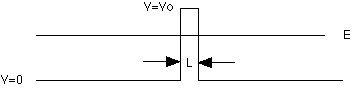# Probability that the electron will pass through the barrier?

Gee Wiz

## Homework Statement

An electron of energy E = 2.2 eV is incident on a barrier of width L = 0.7 nm and height Vo = 3.2 eV as shown in the figure below. (The figure is not drawn to scale.)What is the probability that the electron will pass through the barrier?
The transmission probability is?

## Homework Equations

T=Ge^(-2kL)
G=16(e/v)(1-e/v)
k=sqrt(2m/(h/2∏)^2(U-E)

## The Attempt at a Solution

So I thought this was straight forward and was putting all the values into the formulas and I keep getting 0. Which is not correct...is there something I am overlooking?

Gee Wiz
I got G = 3.4375 eV
k= 5.125e9 J

Am I missing that T is just a coefficient and I should use it as something else..?

Last edited:
Staff Emeritus
Homework Helper
The units you have for G are wrong, though the number is right. The units of ##k## should be 1/length, and it's way off numerically.

It's useful to memorize certain combinations of constants:
\begin{align*}
m_e c^2 &= 511000\text{ eV} \\
\hbar c &= 197\text{ eV nm}
\end{align*} where ##c## is the speed of light. Your expression for ##k## can be rewritten slightly by introducing convenient factors of ##c##:
$$k = \sqrt{\frac{2m_e(U-E)}{\hbar^2}} = \sqrt{\frac{2(m_ec^2)(U-E)}{(\hbar c)^2}}$$

Last edited:
Gee Wiz
Okay, that makes sense so for k I get 5.131. So then I took
T=3.4375e^(-2*5.131*.7)

Gee Wiz
I think I am still missing something, because I keep getting .002607 at my T, yet this is not correct

Last edited:
Homework Helper
Gold Member
here's a nifty site for this. Their formula is correct, I think it's the same as yours.

HOWEVER: they also have a calculator and when I inputed your parameters I get that the probability is 1.0!

I don't know if it's right or wrong, but there it is.

http://hyperphysics.phy-astr.gsu.edu/hbase/quantum/barr.html

Staff Emeritus
Homework Helper
How do you know it's not correct?

Gee Wiz
here's a nifty site for this. Their formula is correct, I think it's the same as yours.

HOWEVER: they also have a calculator and when I inputed your parameters I get that the probability is 1.0!

I don't know if it's right or wrong, but there it is.

http://hyperphysics.phy-astr.gsu.edu/hbase/quantum/barr.html

I tried that, but unfortunately it was not correct. But thank you for the suggestion.

Gee Wiz
I know it is not correct because the online homework marks it incorrectly...but maybe the system is having issues..?

Staff Emeritus